# Determining Enzyme Kinetics of Chymotrypsin with Stopped Flow Spectroscopy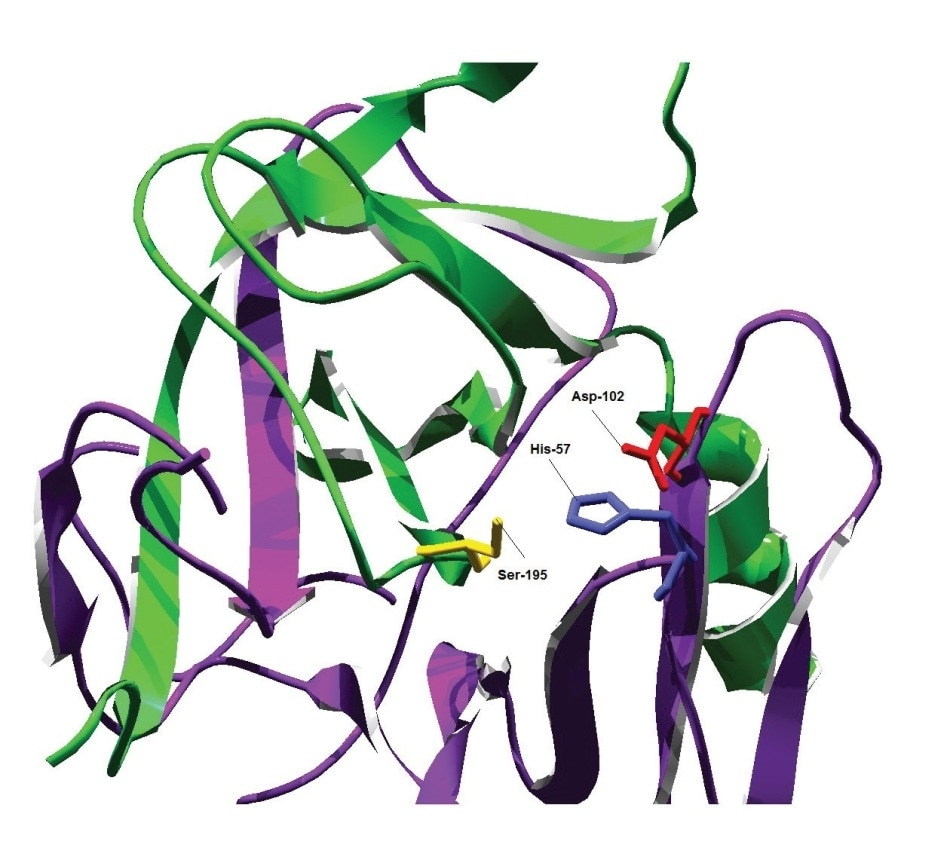Figure 1. Structure of the active site of α-chymotrypsin.

For over 30 years, Applied Photophysics Ltd has manufactured stopped-flow systems to measure enzyme kinetics that have been referenced in numerous scientific publications. The following article demonstrates the use of an SX20 stopped-flow spectrometer to determine the pre-steady-state kinetics of the hydrolysis of p-Nitrophenyl acetate, an enzymatic reaction that is catalyzed by α-chymotrypsin. This enzyme belongs to a class of serine protease enzyme that is present in every aspect of life, as they play an important role in peptide bond hydrolysis. To perform their catalytic activity, α-chymotrypsin and all other serine proteases act at on their active site that contains a catalytic triad. This catalytic triad will typically be composed of aspartic acid, histidine and serine residues, as shown in Figure 1.

The serine residue of a serine protease acts as a nucleophile to target the carbonyl group, whereas the aspartic acid and histidine residues are directly involved in the bonding and transfer of hydrogen molecules. Figure 2 illustrates the steps involved in the catalyzed hydrolysis of p-Nitrophenyl acetate by trypsin and α-chymotrypsin, whereas Figure 3 summarizes the enzyme kinetics of this reaction.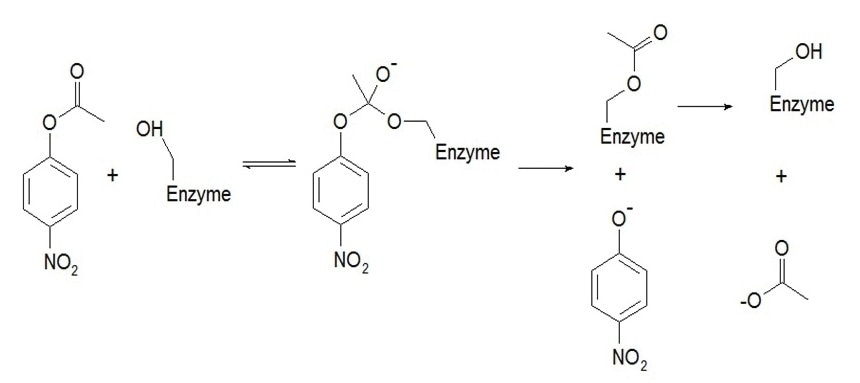Figure 2. The catalyzed hydrolysis of p-Nitrophenyl by α-chymotrypsin.

The catalyzed hydrolysis of p-Nitrophenyl by α-chymotrypsin begins with the nucleophilic serine residue on the active site of α-chymotrypsin attacking the carbonyl group of p-Nitrophenyl acetate. This first step of the reaction is pre-equilibrium and takes place relatively faster than the final two steps. Following the loss of p-Nitrophenolate, a tetrahedral intermediate is formed that allows spectroscopic analysis of this process to be studied at a wavelength of 400 nm. The acetyl-enzyme intermediate is then deacylated to form acetate, as well as a regenerated form of the enzyme, α-chymotrypsin.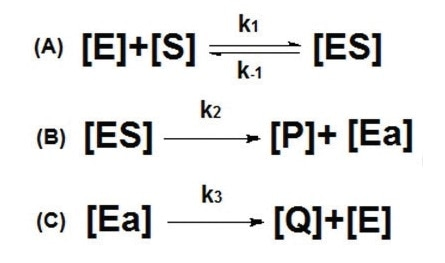Figure 3. Enzyme kinetics of the hydrolysis of p-Nitrophenyl acetate by α-chymotrypsin, where [E] represents the free α-chymotrypsin enzyme, [S] represents p-Nitrophenyl acetate, [ES] represents the tetrahedral intermediate formed in the first step of the hydrolysis reaction, [P] represents the first product p-Nitrophenolate, [Ea] represents the acylated intermediate and [Q] represents the final product of acetate.

## Materials and Methods

All reactions in this experiment were performed using the Applied Photophysics SX20 stopped-flow spectrophotometer that was equipped with a 20 µL optical cell at a pathlength of 2 mm. The light source of the SX20 was a 150 W ozone-free xenon lamp. Absorbance was measured at a wavelength of 400 nm through the use of a standard SX20 photomultiplier tube. For each enzymatic reaction, 1,000 points were recorded over a period of 1 minute, or 60 seconds. All drive volumes in the reaction were 100 µL.

The chemicals used in this reaction were obtained from Sigma-Aldrich and mixed to initiate the enzymatic reaction. These chemicals include:

• p-Nitrophenolate (250 µM to 8 mM, 20% isopropyl alcohol)
• 60 µM α-chymotrypsin (20% isopropyl alcohol, 20 mM phosphate buffer solution (PBS), pH = 7.3)

The drive volumes of these solutions were 200 µL, and the experiment was repeated 6 times to obtain an average of 6 traces. For each concentration, the non-catalyzed reaction was recorded and subtracted from the equivalent catalyzed spectrum. Figure 4 shows the trace of the enzymatic reaction between p-Nitrophenolate and α-chymotrypsin at 400 nm.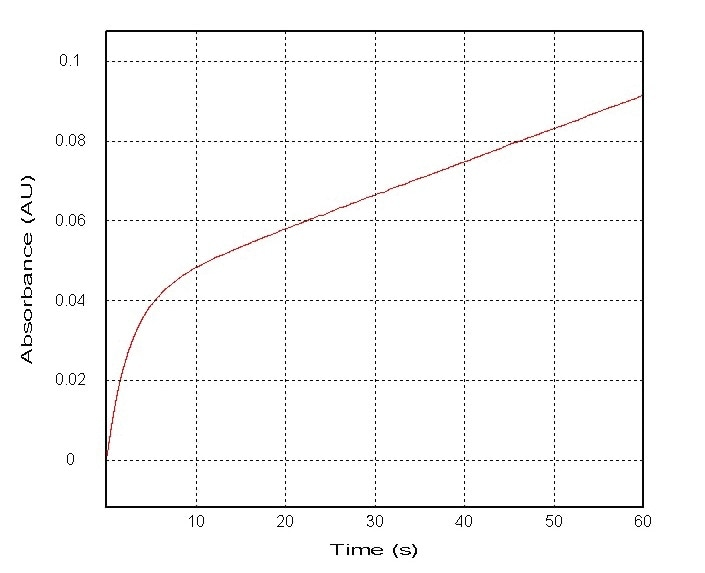Figure 4. Absorbance measurements of the reaction between α-chymotrypsin (60 µM) and a 15 fold excess of p-Nitrphenolate at 400 nm.

## Results

The trace observed following the reaction between 60 µM of α-chymotrypsin and a 100 fold excess of p-Nitrophenolate is shown in the spectrophotometer analysis in Figure 4. The curve in Figure 4 demonstrates that at 0-5 seconds into the reaction, the rate of production is relatively fast, however a linear steady-state rate of production follows the formation of p-Nitrophenolate. The rapid rate of the pre-equilibrium step prevents the ability of the spectrophotomter to adequately detect this trace, therefore the initial traced rate is observed when [ES] is at its highest concentration in the reaction. Since p-Nitrophenolate relies heavily on the second step of this reaction, it accounts for the ‘burst’ phase observed in Figure 4. Furthermore, the shape of the curve also elucidates that the second step of this enzymatic reaction occurs at a faster pace than the final rate limiting deacylation step.

Serine proteases, like most types of enzymes, exhibit saturation kinetics in which the rate of the reaction approaches the hypothesized maximum velocity (Vmax) as [S] increases. Therefore, Vmax describes the rate of the reaction at the point in which the enzyme is completely saturated in the form of [ES]. It can be concluded that the reaction between α-chymotrypsin and p-Nitrophenolate follows the Michaelis-Menten model of enzymatic kinetics, which can be expressed in the equation shown in Figure 5.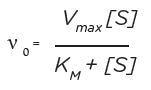Figure 5. The Michaelis-Menten equation, where V0 represents the initial velocity, KM represents the Michaelis-Menten constant that is defined as the concentration of the substrate at which the initial rate of reaction is 50% of the Vmax. The KM constant is often used to compare substrate affinities in enzyme-substrate systems.

Additionally, the rate constant kobs indicates the rate constant of the initial exponential phase of the reaction and is achieved by the following equation: Ae-kt + bt + c. This equation uses the fitting function provided by SX’s Pro-Data Viewer. Figure 6 shows how the substrate concentration can affect kobs.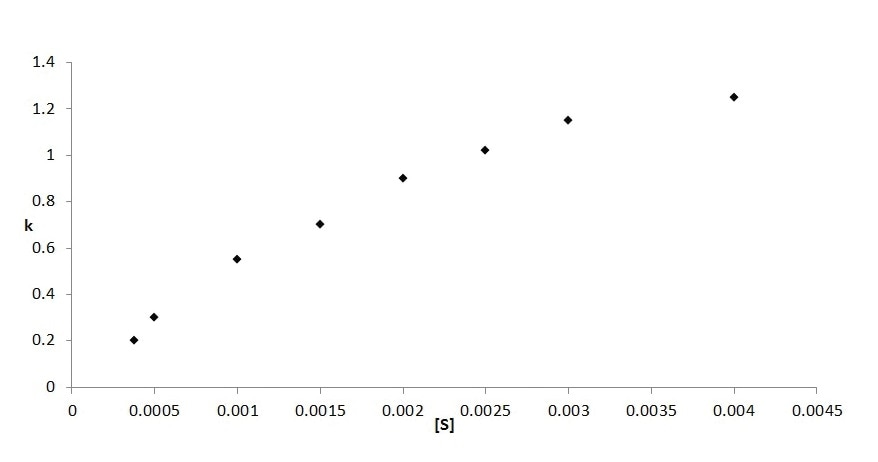Figure 6. The variation of kobs in relation to the substrate concentration involved in the reaction between α-chymotrypsin and p-Nitrophenolate. It can therefore be demonstrated that under the conditions where [S] is greater than [E], the value of kobs is related to the following equation shown in From the kobs equation, the values of KM and k2 can be evaluated by determining the first order rate constant that exists when the initial concentrations of the substrate increase. To obtain a straight line graph in which the slope is equal to KM/k2 and the intercept is equal to 1/k2, a graph that plots 1/kobs against 1/[S] can be utilized. In Figure 8, this technique was used to obtain a Lineweaver-Burk plot of α-chymotrypsin.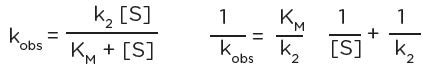Figure 7. The kobs equation.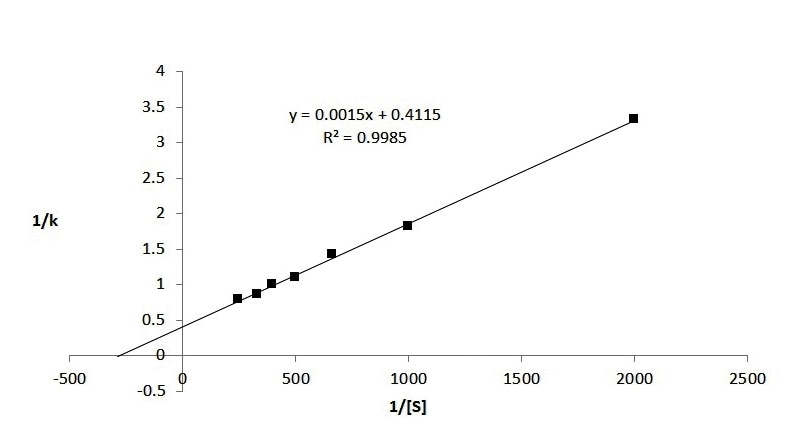Figure 8. Lineweaver-Burk plot of α-chymotrypsin, in which the line of best fit was generated using linear regression. These results are further described in Table 1.

Table 1. Parameters extracted from Figure 5.

Non-Linear Regression Linear Regression
k2 (s-1) 2.38 2.43
KM (10-3 M) 3.40 3.65

Once the reaction reaches the steady state it relies heavily on the third step of the reaction shown in Figure 3 as a result of the relatively slower rates of the first two steps of the equation, thereby making this final step rate determining. Based on the results of the SX20 spectrophotometer analysis, it can be determined that the stead state-rate of the reaction is directly related to the linear part of the trace shown in Figure 4, since the change in absorbance on this graph can be related to the changing concentration levels of p-Nitrophenolate according to the Beer-Lambert Law. Therefore, it can be determined that the burst phase of the reaction is roughly equal to the concentration of the active site. The value of k3 was assessed based on the equation in Figure 9.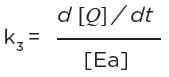Figure 9. The equation to determine the value of k3, which was determined to be 0.016 s-1 from the reaction between α-chymotrypsin and p-Nitrophenolate.

## Conclusion

The Applied Photophysics Ltd SX20 stopped-flow spectrophotometer is a useful tool to analyze the pre-steady-state kinetics of enzymatic reactions. The reaction between α-chymotrypsin and p-Nitrophenolate was studied here to determine the kinetic parameters of this process. While this reaction is relatively slow, the data obtained by the SX20 stopped-flow spectrophotometers can measure kinetics from 0.5 ms following the initial mixing of the reactant chemicals.

## References

1. Gutfreund, H. & Sturtevant, J.M. The mechanism of the reaction of chymotrypsin with p-nitrophenyl acetate. The Biochemical Journal 63, 656-61 (1956).
2. Hedstrom, L. Serine protease mechanism and specificity. Chemical Reviews 102, 4501-24 (2002).
3. Bender, M., Kezdy, F. & Wedler, F. α-Chymotrypsin: Enzyme Concentration and Kinetics. Journal of Chemical Education 44, 84-88 (1967).
4. Gutefreund Kinetics For The Life Sciences. (Cambridge University Press: Cambridge, 1995).
5. Leatherbarrow, R.J. Using linear and non-linear regression to fit biochemical data. Trends in Biochemical Sciences 15, 455-8 (1990).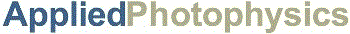This information has been sourced, reviewed and adapted from materials provided by Applied Photophysics.

For more information on this source, please visit Applied Photophysics.

## Citations

Please use one of the following formats to cite this article in your essay, paper or report:

• APA

Applied Photophysics. (2019, January 08). Determining Enzyme Kinetics of Chymotrypsin with Stopped Flow Spectroscopy. AZoNano. Retrieved on May 26, 2019 from https://www.azonano.com/article.aspx?ArticleID=4803.

• MLA

Applied Photophysics. "Determining Enzyme Kinetics of Chymotrypsin with Stopped Flow Spectroscopy". AZoNano. 26 May 2019. <https://www.azonano.com/article.aspx?ArticleID=4803>.

• Chicago

Applied Photophysics. "Determining Enzyme Kinetics of Chymotrypsin with Stopped Flow Spectroscopy". AZoNano. https://www.azonano.com/article.aspx?ArticleID=4803. (accessed May 26, 2019).

• Harvard

Applied Photophysics. 2019. Determining Enzyme Kinetics of Chymotrypsin with Stopped Flow Spectroscopy. AZoNano, viewed 26 May 2019, https://www.azonano.com/article.aspx?ArticleID=4803.

## Tell Us What You Think

Do you have a review, update or anything you would like to add to this article?Leave your feedback
Submit